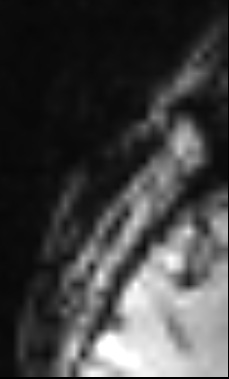# Edit a segmentation programatically in simpleitk

Hello,
I’m very new to itk/simpleitk. What I need to do is:

1. Segment a volume in an image A (I do this in 3D Slicer, manually).

2. In simpleitk, apply the segmentation in another image, B (assume A and B are different frames in a 4D series with no movement between so no registration is needed)

3. Compare the segmented volumes from A and B voxel by voxel and depending on certain properties of their intensities, either keep or remove the corresponding voxel from the initial segmentation.

My questions are:

a) How do I use a segmentation to select a specific volume from a larger volume? I can load the nrrd file as a SimpleITK.Image object and visualise it. How do I “apply” it on the new volume to extract a new sub-volume? (sorry if this is a very basic question)

b) How do I edit a segmentation by removing specific voxels programatically?

If these are covered in an existing tutorial, please point me to the right resource.

Hello @kliron,

This is more of a numpy answer, but hopefully this does what you were thinking of (not sure):

``````impor SimpleITK as sitk
import numpy as np

#Potentially resample image_b onto image_'s grid
#image_b = sitk.Resample(image_b, image_a)
#image_a_view = image_a.GetArrayViewFromImage(image_a)
#image_b_view = image_b.GetArrayViewFromImage(image_b)
#segmentation_arr = segmentation.GetArrayFromImage(segmentation)

image_a_view = np.array([[2,3],[4,5]])
image_b_view = np.array([[6,6],[7,7]])
segmentation_arr = np.array([[0,1],[1,0]])

remove_condition = list(np.logical_and(image_a_view[segmentation_arr==1]>2,
image_b_view[segmentation_arr==1]==6))
if rc:
segmentation_arr[mi] = 0
new_segmentation = sitk.GetImageFromArray(segmentation_arr)
new_segmentation.CopyInformation(segmentation)
``````
1 Like

Thank you Ziv, I will try to implement this approach. I am still unclear on how to extract a sub-volume from the initial 3D volume by using a segmentation. I looked into the RegionOfInterestImageFilter but if I understand correctly this can only define rectangular ROIs and that will not do for what I need to do.

Hello @kliron,

What do you mean when you say “extract a sub-volume”?

As the basic structure of an image is a rectangular grid, no matter what you want, you’ll get a rectangle. The question is, what do you want that rectangle to be and what do you want it to contain. You can easily zero out all of the pixels/voxels outside the mask.

It would help if you provided some drawings to illustrate what it is you are trying to do.

Here is an example.
This is a slice of a 3D volume where I’ve segmented the choroid plexus in slicer. From python and simpleitk, I load the segmentation and the original volume and create a LabelOverlay which results in the image you see below. My goal in the first part of my problem is to get the actual voxel intensities of the choroid plexus from the initial image as a separate volume that does not contain anything else but those voxels. I tried to go by way of defining a `shape` by using my segmentation as the input of LabelShapeStatisticsImageFilter and then getting a RegionOfInterest using the initial volume and this shape but what I get is a rectangular volume that contains brain tissue, not plexus.

Here is one single slice from the overlay volume:

Here is my code:

``````
shape = sitk.LabelShapeStatisticsImageFilter()
shape.ComputeOrientedBoundingBoxOn()
shape.Execute(segm)
result = sitk.RegionOfInterest(orig, shape.GetRegion())
``````

The resulting region not only is a rectangular volume (not the complex anatomy I segmented), it seems to also be completely wrong, does not contain any of the plexus volumes on either side, just skull and some brain parenchyma. Here is a slice:Hello @kliron,

Please try this (don’t forget to set the `label_of_interest` to the correct value):

``````segm = sitk.ReadImage(os.path.join(segmentations_dir, 'Plexus.nrrd'))
shape = sitk.LabelShapeStatisticsImageFilter()
shape.Execute(segm)
bounding_box = shape.GetBoundingBox(label_of_interest_value)
# The bounding box's first "dim" entries are the starting index and last "dim" entries the size
result = sitk.RegionOfInterest(orig, bounding_box[int(len(bounding_box)/2):], bounding_box[0:int(len(bounding_box)/2)])
``````

The code above will yield an image that bounds the segmentation, crop-to-segmentation.

If you just want to get the actual voxel intensities of the choroid plexus, a list of the intensity values, then you don’t need the operation above. Just use the code previously posted (i.e. `image_a_view[segmentation_arr==label_of_interest_value]` will give you the list of values).

Hello @Ziv,
Your solution works perfectly. Thank you!